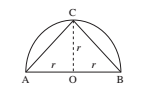# SSC CGL 2018 Practice Test Papers | Quantitative Aptitude (Day-10)

Dear Aspirants, Here we have given the Important SSC CGL Exam 2018 Practice Test Papers. Candidates those who are preparing for SSC CGL 2018 can practice these questions to get more confidence to Crack SSC CGL 2018 Examination.

[WpProQuiz 2786]

Click “Start Quiz” to attend these Questions and view Explanation

1). The value of (16)2x (28)2/ (64)2 is

1. 59
2. 56
3. 78
4. 49

2). In SI, a sum of money becomes 5 times the principal amount in 16 years. What is the rate of interest?

1. 25%
2. 18%
3. 16%
4. 17%

3). The sum of three numbers is 168. The ratio of second and the third number is 7: 9 and the 1st and the third are as 8: 27, what is second number?

1. 65
2. 45
3. 63
4. 72

4). A can complete a work in 40 days while B can do it in 25 days. A started the work and after 8 days A left the job. After how many days B will complete the remaining work?

1. 160/13
2. 28
3. 20
4. 200/13

5). Harshitha and Harija can do a piece of work in 20 days. Harini who is twice effient of harshini can do a piece of work in 5 days. Find the time taken by Harshitha to do the work?

1. 10
2. 20
3. 28
4. cannot be determined

6). 50 workers can complete a job in 6 days working 8 hours a day. If 40 workers are employed to complete the job in 20 days, and then find the number of hours they work per day to complete the job?

1. 4 hours
2. 6 hours
3. 9 hours
4. 3 hours

7). The number of straight lines that can be drawn in a plane with 21 given points, assuming that no three of them are collinear is—

1. 210
2. 46
3. 2·23!
4. 21!

8). Area of the largest triangle drawn in a semicircle of radius r will be—

1. r2
2. r3
3. 2r2
4. 2r3

9). If 25% of 3/ 4 of 4 /5 of a number is 66, what would be the value of 25 percent of that number?

1. 1
2. 110
3. 10
4. 20

10). A sum of money was distributed among 400 men and some ladies in the ratio of 10: 9. If each man got Rs. 5 and a lady Rs. 4. What is the total number of ladies?

1. 220
2. 200
3. 190
4. 210

(16)2x (28)2/ (64)2 = 49

Let principal = Rs. x

∴ Amount a. = 5x

S.I. = A – P

S.I. = 5x – x = Rs.4x

4x =x*16*r/100

Rate% = 25%

Ratio of second number: third number= 7:9=21:27

Ratio of first number: third number    = 8:27

Ratio of three numbers= 8:21:27

8x+21x+27x=168

X=3

Second number=21×3=63

One day work of A=1/40

One day work of B=1/25

In 8 days A completed 8*1/40= 1/5 work

Remaining 4/5 of the work was completed b y B

4/5*25=20 days

x = 50 × 6 × 8 /40 × 20 = 3 hours

21C2=21×20/2=210

Perpendicular height of the largest triangle drawn in a semi-circle of radius r = r∴ Area of the largest possible triangle = 1/ 2 (base × height) = 1/ 2 (2r) × (r) = r2

Let the number be x

Then, x × 4/ 5 × 3/ 4 × 25 /100 = 66

⇒ x = 66 × 20 /3 = 440

∴ 25 per cent of the number = 440 × 25/ 100 =110

Let the number of women be x

400 × 5 /(x × 4) = 10 /9

400 × 5 × 9 = 10 × x × 4

∴ x = 400 × 5 × 9/ (10× 4 )= 450